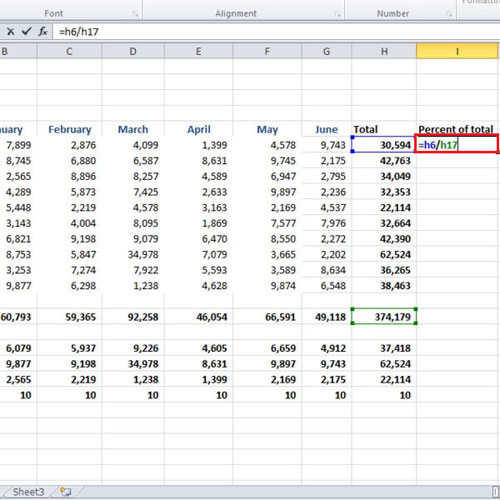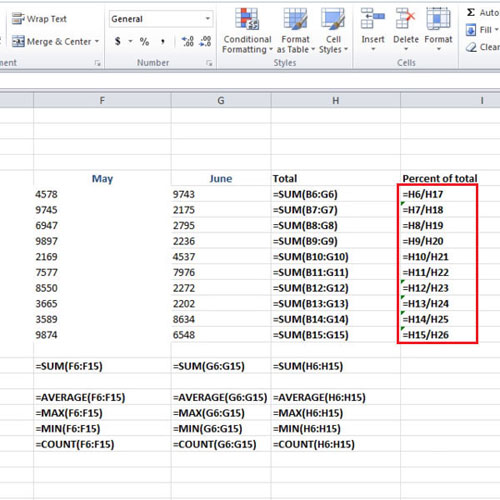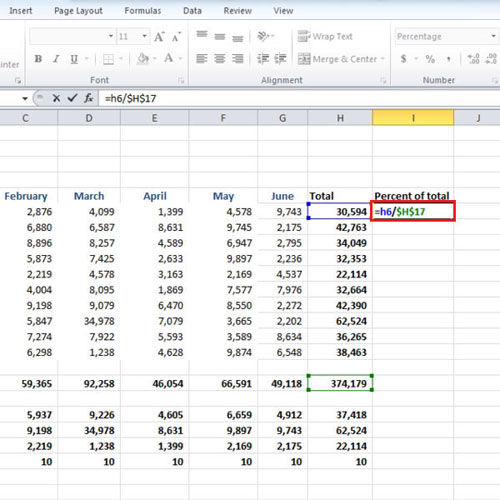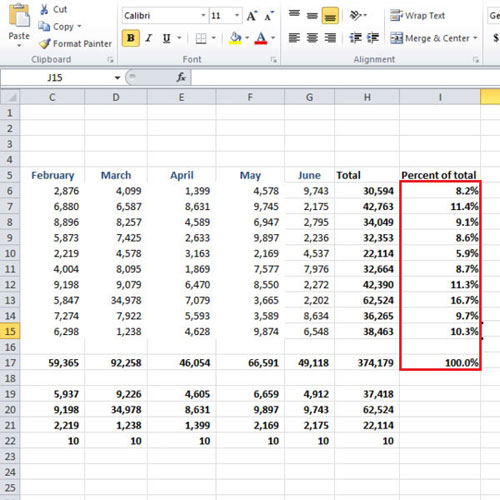## How to Prevent Errors Using Absolute References in Excel 2010

Handling data in excel can be difficult sometimes. There is always a room for errors and these errors come in different ways. In this tutorial, we will have a look as to what absolute reference is and how can we use it to prevent errors. When you are doing some calculations, you give cell references and when you use the auto fill feature, Excel guesses the pattern and tries to use the same formula or in the same way the previous value was calculated. However, this may sometimes give reference errors.
Follow this step by step tutorial to learn how to prevent errors using absolute references in Ms Excel.

Step # 1 – Take out the percentage
In this worksheet, we have 10 cities and the sales values are given for six months i.e. from January till June. The total of each month’s sale is calculated and a grand total is calculated in cell “H17”. Consider, we need to find out what percentage did a particular city contribute to the total number of sales in 6 months. In order to do that, we will divide the total value of sales of a city by the grand total. Under the “percentage of total” titled, write “=h6/h17”. Press the “enter” key when done and the percentage will be calculated.Step # 2 – Use auto fill to take out the rest of the percentages
After the first percentage is taken out, click on the “%” button which is there in the “number” group. Use the decimal button to increase or decrease the decimal places. Use the auto fill to calculate the rest of the percentages. It will show you errors; press the “ctrl + `” key to check for errors. We divided cell “h6” by cell “h17” so we wanted the next formula to be “h7” divided by “h17”. However, this is not the case over here.
Ms Excel jumped from “h17” to “h18” when it was asked to calculate the percentage of cell “h7”.Step # 3 – Use absolute reference
In order to prevent such errors from happening, we should use the absolute reference. This would allow the cell reference to be the same, no matter which cell you are calculating the formula in. Write “h6/h17” in the percent of total column. Before pressing the “enter” key, press the “F4” key and it will turn the last cell reference which is cell “h17” into an absolute reference. The dollar sign will appear before the letter “h” and the number “17”. This will ensure that absolute reference has been established.Step # 4 – Drag the auto fill handle
Once you have made the absolute reference, press the “enter” key and after that drag the same formula down till the last city. No errors will be shown. Press “ctrl +`” to confirm that cell “h17” has been used to take out the rest of the percentages.